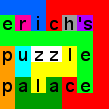Puzzles of the Week

The following puzzles were chosen as puzzle of the week at MathPuzzle.com. All puzzles copyright Erich Friedman, 1999-2013.

1. Form a sequence of digits by starting with 1 and 2, and letting each the next digit(s) be the product of the two previous digits: 122483224648242432168881262648646482... Show that the numbers 0, 5, 7, and 9 never appear, but arbitrarily long sequences of 8's appear.
2. Divide a triangle with sides 21, 42, and 42 into 6 similar triangles with different sizes.
3. This is a rolling block maze. There is a 2×2×1 slab that rolls along the board avoiding obstacles. It starts at SS and should finish at FF.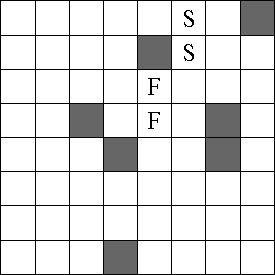4. What's the smallest square that can be tiled with 2 or more integer-sided squares so that equal sized squares don't touch? What's the smallest rectangle with this property?
5. A saturated triangle packing is a collection of non-overlapping triangles sothat each vertex of each triangle touches exactly one other vertex of an another triangle. Find a saturated triangle packing with 12 equilateral triangles of two different sizes, and of 10 equilateral triangles of 3 different sizes.
6. This is the 12345 maze, in which you start on S. You can move 1 square in any direction, followed by 2 squares in any direction, then 3, then 4, then 5, and back to 1 again until you land on F.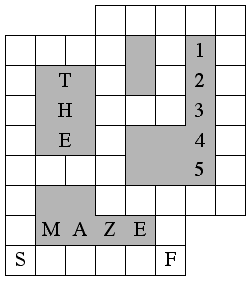7. The dots below are located at the corners of a collection of squares. No two squares can share a corner. What are the squares?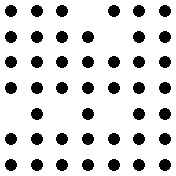8. There are 24 different ways that 0-6 unit holes can be cut in a 2×3 rectangle. Arrange these 24 different rectangles in a 12×12 grid so that all the holes are contiguous.
9. Cut an 11×11 square into integer-sided rectangles with areas in the teens.
10. A number that is the reverse of the sum of its proper substrings is 941, since 94 + 41 + 9 + 4 + 1 = 149. Find other numbers with this property.
11. This is another rolling block maze. Roll the U from START to FINIS. it must avoid the obstacles but the hole can roll over them.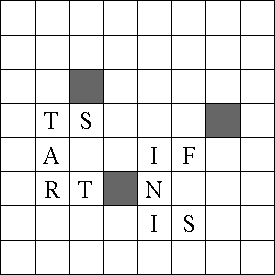12. A slightly larger rolling U maze.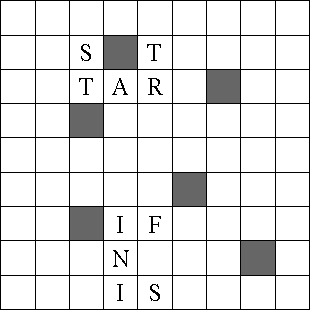13. This is a rolling block maze with 3 different blocks shown in red. They cannot change levels, and must always be completely supported. Get the upper most block above F.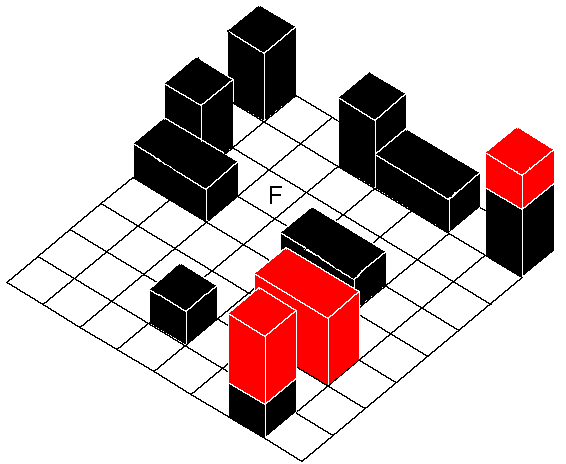14. Using a word search format, fit the words ONE through EIGHT into a 5×5 square, so that the numbers read horizontally, vertically, or diagonally. Also, fit ONE through TWELVE into a 6×6 square.
15. Take a tour of the lower 48 states, always moving from a state to an adjacent state, and never visiting a state twice. If you start in MAINE, visit MARYLAND 16th, WASHINGTON 31st, KANSAS 39th, and end in CALIFORNIA, in what order did you visit the states?
16. What's the fewest number of convex pentagons that a triangle can be dissected into? How about the fewest number of convex pentagons in a square?
17. Here's another state tour puzzle.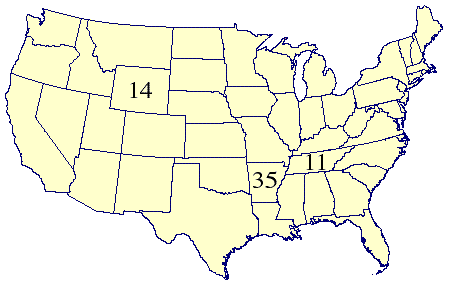18. What are the best packings of 1 to 5 circles on a torus?
19. A state meta-puzzle: what is the fewest number of states you have to name in a state tour to have a unique solution?
20. A new years puzzle for 2002. Place 4 horizontal dominoes (covering adjacent squares) on the ring below so that there are exactly 2002 ways to tile the remaining area with dominoes.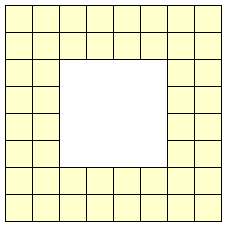21. Complete this number crossword. No number starts with 0.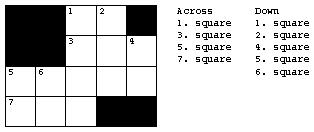22. Tile a 140×140 square with 4 squares each of size 1, 2, 3, . . . 24.

23. Notice that A = 275 = 14348907, B = 395 = 90224199, and C = 12395 = 2919823044470199, A and B have the same number of digits, and C contains exactly the digits of A and B together. What are the smallest fourth powers with this property?

24. Divide this square into polyominoes along the grid lines so that each polyomino contains exactly one circle that is its center of rotational symmetry.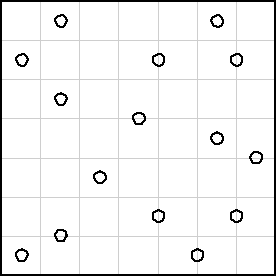25. The squares 69696, 56722567225, and 95540955409 each repeat their digits with at least two full periods. What are the next squares with this property?

26. Find a collection of equal triangles with the property that every point that is the vertex of a triangle is the vertex of exactly 3 triangles.

27. Take an 18×18 grid of squares, and remove two of them so that the resulting figure contains exactly 2002 squares of all different sizes made from the remaining squares.

28. Divide a 20×20 (or 23×23) square into 9 squares or dominoes with sides 1-9. Divide a 25×25 square into 10 squares or dominoes with sides 1-10.

29. The 12 pentominoes have been paired up in the figure below. Divide them up again.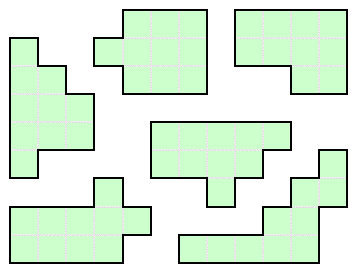30. Arrange 14 cubes so that each cube touches 6 other cubes along some portion of a face.
31. Dissect a square into 7 dominoes of 3 different sizes. Then dissect a square into 7 straight trominoes of 3 different sizes.
32. Find a 4×4 matrix whose entries are distinct positive integers less than 30 whose row SUMS are the same, and whose column PRODUCTS are the same.
33. 4, 1, 1, and 42025, 14641, 10000 are examples of triples of squares whose digits add componentwise to 666...6. Find another such triple.
34. Sometimes a day is the sum of the digits of the horizontally and vertically adjacent days on that month's calendar. For example, this happened on December 14, 22, 24, and 26 of 2002. There is only one day in 2003 on which this will happen but be the ONLY time that month it will happen. When is it?
35. Pack the 21 a×b×c integer-sided blocks with sides 0<a<b<c<7 into a 7×7×15 block.
36. Write 2003 as the sum of distinct positive integers with the same digits.
37. Arrange the digits 2, 3, 4, 5, 6, and 7 in a 2×3 matrix so that the product of the two 3-digit numbers across is equal to the product of the three 2-digit numbers down.
38. Divide a 67×67 square into squares of size at least 10.
39. Find positive integers A, B, C, D, and E all less than 100 so that A2 + B2 + C2 = D2 + E2 and A3 + B3 + C3 = D3 + E3.
40. Start with 2003. Chop it up any way you like: 20:0:3. Square the pieces and add them together to get a new number 400 + 0 + 9 = 409. Repeat until you get 2004. Find a 6-step path from 2003 to 2004. Then find a 4-step path from 2003 to 2004.
41. Find an expression for 2004 using the fewest number of identical digits, addition, subtraction, multiplication, division, and exponentiation.
42. 722855133 has the peculiar property that all of its length 3 substrings are divisible by 19. Find the largest number so that all length 2 substrings are distinct and divisible by 19. Find the largest number so that all length 4 substrings are distinct and divisible by 19.
43. Find a 83-omino that contains exactly 2004 rectangles of various sizes.
44. For each puzzle below, find the starting square and draw a path moving horizontally and vertically that passes through each open square exactly once. For each segment in the path, you must go as far as possible, changing direction only when you are blocked by the grid's edge, a black square, or a square already visited.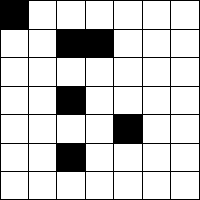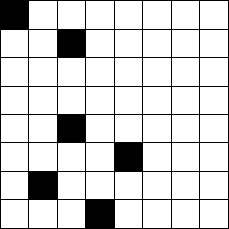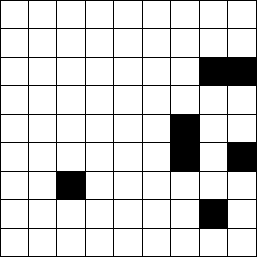45. Hex versions of the same concept: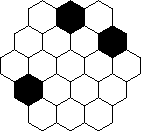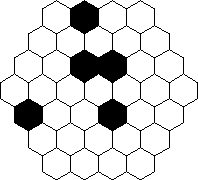46. More of the same: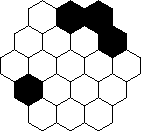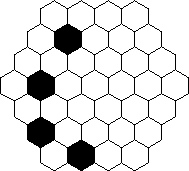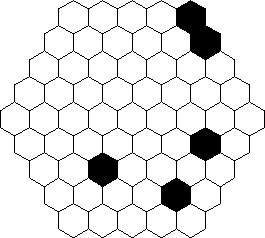47. Divide a square into L tetrominoes in two different ways so that each L touches exactly 4 other L's.
48. More path puzzles. But this time the path has to go through each yellow square twice (one horizontally and once vertically) and you have to turn at the green squares.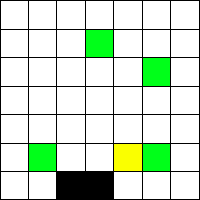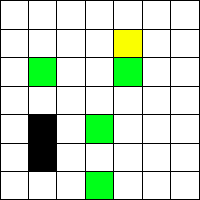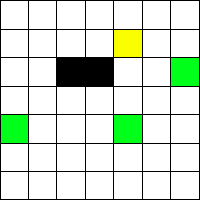49. What do these polyforms have in common?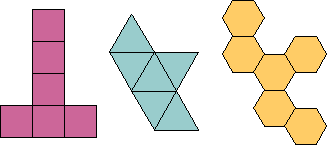50. If you cut a square in half along a diagonal, you get a tan. What is the side of the smallest tan that contains 3 non-overlapping tans of side 1?

51. Find the 6 missing digits that make the equation (x^x + xx) / xx = 2005 true.

52. Find 12 points in the plane, so that exactly 3 points are "hidden" from each point.

53. Insert some of the symbols + – × / ^ ( and ) to the string of digits 8 7 6 5 4 3 2 to make the result equal to 2006.

54. Place one more orange square so that there are exactly 2006 ways to fill the remaining area with squares.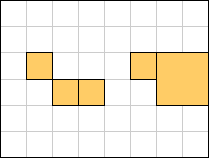55. A moderator writes two non-zero digits on a piece of paper. He tells P the product of the two digits, and he tells S the sum of the two digits. P and S then have the following conversation:

P says "I don't know the digits".
S says "I don't know the digits".
P says "I don't know the digits".
S says "I don't know the digits".
P says "I don't know the digits".
S says "I don't know the digits".
P says "I don't know the digits".
S says "I don't know the digits".
P says "I know the digits".

What are the digits?

56. Draw a graph isomorphic to the cube where every edge crosses exactly two (nonadjacent) edges.

57. Using the rules below, one at a time, on some consecutive substring, get from {2,2,2} to {1,2,1,1,1} in 13 moves. You never need a string longer than 6 digits.

Rule #1: {2,1,2} → {1,1}
Rule #2: {2} → {1,2,2}
Rule #3: {1,1} → {2}

58. Add the same polyomino to both of these shapes to get the same shape.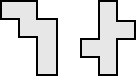59. What is the only positive integer n with the property that together 4n and 5n use each digit 1-9 exactly once?

60. Using the digits 3, 4, 5, 6, 7 each once, and the usual + – × / ^ ( ) and concatenation, make the new year 2007, in two different ways.

61. Place 4 white knights and 4 black knights on a 6×5 chessboard so that each knight attacks 3 knights of the opposite color and none of its own color.

62. Using only + – × / ^ ( ) and decimal points:

a) approximate π=3.14 to three significant figures with three 2's,
b) approximate e=2.718 to four significant figures with four 7's,
c) approximate γ=.5772 constant to four significant figures with four 8's.

63. Using each of the following collections of 5 digits, along with the usual arithmetic signs + – × / ^ ( ), make a total of 2008:

{1,3,8,8,8}, {1,4,4,4,8}, {2,3,8,8,8}, {2,5,9,9,9}, {2,7,8,8,8},
{2,8,8,8,9}, {3,4,8,8,8}, {4,4,4,8,9}, {4,5,8,8,8}, {5,6,6,6,8}.

64. Connect pairs of houses with straight roads so that only two different distances are created.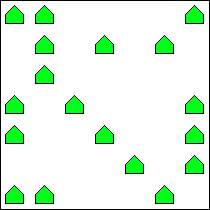65. Everyone knows that 24 = 42. But hardly anyone knows 25 + 27 + 29 + 53 + 54 = 52 + 72 + 92 + 35 + 45. Find two more equations like this: each side being the sum of single digits to a single digit power, with no terms repeated.

66. Cut the ornament into 24 equal shapes. Four of the shapes have already been cut to start you off.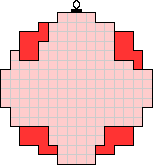67. Put single digits in each box so that a) the equation is true and b) the fulcrum balances if the digits are the weights of the boxes.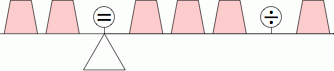68. Write 2009 using six 7's. You may use as many of the arithmetic symbols + – × ÷ ^ ( ) as you like.

69. Decorate the tree below with the 5 different sizes of ornaments shown. The ornaments should cover the entire tree and not overlap. You should use only 17 ornaments, including at least one of every size, and no yellow ornament should touch a blue ornament.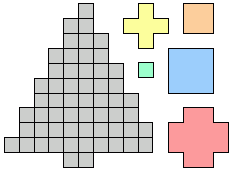70. Start at 2011. By moving through the maze and doing any arithmetic operations you encounter, exit the maze with a result of 2012. You may pass through an operation several times, but not twice in a row.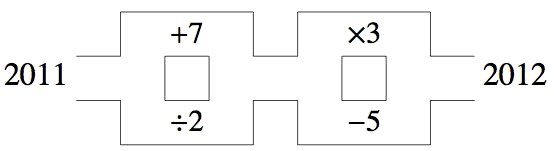71. Put the digits 1 through 5 into five of the circles below. If a circle contains the digit n, then the circles that are n clockwise and n counterclockwise from that circle should also contain digits.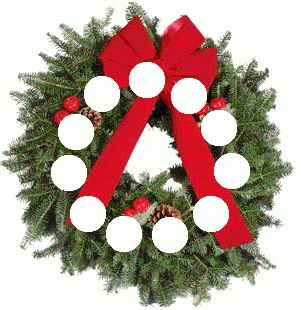Back to